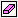# MultiPlot Graph

Plot results of multiple simulations

• Library:
• Robust Control Toolbox

•## Description

The MultiPlot Graph block displays signals in a MATLAB® figure. Each time you simulate the model, the block adds a new line to the figure, cycling through seven colors. This block allows you to view the results of multiple simulations on a single MATLAB figure. For instance, you can use the block with the Uncertain State Space block to visualize Monte Carlo and worst-case simulation time responses.

The input signal can be scalar or vector. If the input signal is a vector, then the block plots each component of the vector in separate axes.

To export the visible plot data to the MATLAB workspace, in the figure window, click. The block exports the data in a variable having the name you specify in the Variable for Export to Workspace parameter.

To clear the data from all axes, in the figure window, click.

## Ports

### Input

expand all

Providing a scalar signal creates one plot. To create multiple subplots, combine the signals you want to plot into a vector signal, using blocks such as:

## Parameters

expand all

The t-min and t-max parameters set the x-axis limits. To set different lower limits for each subplot, set t-min to a vector of the same dimensions as the input signal.

#### Programmatic Use

 Block Parameter: `tmin` Type: scalar, vector Default: 0

The t-min and t-max parameters set the x-axis limits. To set different upper limits for each subplot, set t-max to a vector of the same dimensions as the input signal.

#### Programmatic Use

 Block Parameter: `tmax` Type: scalar, vector Default: 20

The y-min and y-max parameters set the y-axis limits. To set different lower limits for each subplot, set y-min to a vector of the same dimensions as the input signal.

#### Programmatic Use

 Block Parameter: `ymin` Type: scalar, vector Default: –1

The y-min and y-max parameters set the y-axis limits. To set different upper limits for each subplot, set y-max to a vector of the same dimensions as the input signal.

#### Programmatic Use

 Block Parameter: `ymax` Type: scalar, vector Default: 1

Specify the sample time of the block. A sample time of –1 means that the block inherits its sample time from the upstream blocks.

#### Programmatic Use

 Block Parameter: `Tsamp` Type: scalar Default: –1

Specify the title of the MATLAB figure that the block generates.

#### Programmatic Use

 Block Parameter: `titleString` Type: character vector Default: `''`

To export the plot data to the MATLAB workspace, in the figure window, click. The block exports the data to a variable with the name you specify in this parameters. The data is saved in a `struct` array, following the behavior of a To Workspace (Simulink) block with its Save format parameter set to `Structure With Time`.

#### Programmatic Use

 Block Parameter: `vnamesave` Type: character vector Default: `''`

## Version History

Introduced in R2007a